# Bi-Directional Resistor Color Code Calculator

 resistance calculator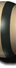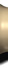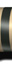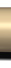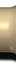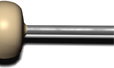K M ±5% ±10% ±20%

Bi-Directional Resistor Color Code Calculator: Calculates resistance from colors and colors from resistance, each with image-based output. Both color and numeric interfaces function as input and output.

## Resistor Band Colors

Most often, the resistance presents itself with colored rings around it. Each color corresponds to a number. The correspondence between the numbers and the colors of the rings constitutes what is called the color code and makes it possible to determine the value of a resistor as well as its tolerance.

## How to calculate the value of a resistance?

To know the value of an electrical resistance (in ohms Ω), there is an ohmeter , or the classic method is based on reading the color code drawn on resistance.

The international standard IEC 60757, titled Color Designation Code (1983), defines a color code to mark their value on resistors, capacitors and other components.

## What do the different colors of resistance bands mean?

 Colour Digit Multiplier Tolerance Black 0 1 Brown 1 10 ± 1% Red 2 100 ± 2% Orange 3 1000 Yellow 4 10000 Green 5 100000 ± 0.5% Blue 6 1000000 ± 0.25% Violet 7 10000000 ± 0.1% Grey 8 ± 0.05% White 9 Gold 0.1 ± 5% Silver 0.01 ± 10% None ± 20%

## 4-ring resistors

The first two rings give the significant figures (the first gives the ten and the second the unit).

The third gives the multiplier (the power of 10 that must be multiplied with the significant figures).

The fourth is the tolerance (the uncertainties on the real value of the resistance given by the manufacturer).

## 5-ring resistors

The first three rings give the significant figures.

The fourth gives the multiplier (the power of 10 that must be multiplied with the numbers significant).

The fifth is the tolerance (the uncertainties on the real value of the resistance given by the manufacturer).

## 6-ring resistors

The first four rings have the same meaning as the 5-ring resistors (see above).

The sixth is a temperature coefficient (variation of the electrical conductivity with thetemperature).

## Why is there a difference between the theoretical value and the measured value?

The measured value is never exact but must be within the tolerance interval of the electrical resistance.

## How to know the reading direction of the resistance color code?

Most often the first ring is closest to one end. Also, the tolerance ring is sometimes separated by a slight space from the other rings (sometimes it ends up on the resistance bump).

Share it